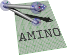amino  1.0-beta2 Lightweight Robot Utility Library
planar.hpp File Reference

Object types for SE(2) representations. More...

#include <complex>

Go to the source code of this file.

## Classes

struct  amino::BaseCmplx
Base type for complex numbers and 2D vectors. More...

struct  amino::Vec2
A 2D vector represented using a complex number. More...

struct  amino::AngleP
A planar rotation angle. More...

struct  amino::Cmplx
A complex number. More...

struct  amino::RotMatP
A planar rotation matrix. More...

struct  amino::CmplxTran
Planar transformation represented with a complex number and translation vector. More...

struct  amino::TfMatP
A Planar transformation matrix. More...

amino
amino namespace

## Functions

static aa_tf_vec2 amino::operator* (const Cmplx &c, const Vec2 &v)
Rotate a 2D vector using a complex number.

static aa_tf_vec2 amino::operator* (const aa_tf_rotmatp &R, const Vec2 &v)
Rotate a 2D vector using a rotation matrix.

static aa_tf_rotmatp amino::operator* (const aa_tf_rotmatp &R1, const aa_tf_rotmatp &R2)
Multiply (chain) two rotation matrices.

static aa_tf_vec2 amino::operator* (const aa_tf_tfmatp &T, const aa_tf_vec2 &p)
Transform a 2D vector using a transformation matrix.

static aa_tf_vec2 amino::operator* (const aa_tf_cv &E, const aa_tf_vec2 &p)
Transform a 2D vector using a complex number and translation vector.

static aa_tf_tfmatp amino::operator* (const aa_tf_tfmatp &T1, const aa_tf_tfmatp &T2)
Multiply (chain) two transformation matrices.

static aa_tf_cv amino::operator* (const aa_tf_cv &E1, const aa_tf_cv &E2)
Chain two complex-numbers and translation vectors.

## Detailed Description

Object types for SE(2) representations.

The class definitions in this file provide convenience methods to simplify type conversions between various orientation and transformation representations. The underlying operatations (and many others) are declared in tfp.h.